# CAT Quant Mock Test - 1

## 25 Questions MCQ Test CAT New | CAT Quant Mock Test - 1

Description
Attempt CAT Quant Mock Test - 1 | 25 questions in 35 minutes | Mock test for CAT preparation | Free important questions MCQ to study CAT New for CAT Exam | Download free PDF with solutions
QUESTION: 1

### The cost of one helmet, two pairs of gloves, three pairs of pads, four bats and five balls increased by 11%, 22%, 33%, 44% and 55% respectively, over that of the previous year. Last year, if I had spent equal amounts purchasing each of the given varieties of items (i.e., helmets, gloves, pads, bats and balls), by what percentage would the total cost of all the items I purchased increase this year, over that in the previous year?

Solution:

Let the amount that spent on each variety of items by x.

Hence, total amount I spent last year = 5x.

This year I would have spent x (1.11) + x (1.22) + x (1.33) + x (1.44) + x (1.55)

Hence the total cost increased by

x x 0.11[1 + 2 + 3 + 4 + 5] = x x 0.11 x 15

∴ Percentage increase in cost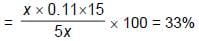QUESTION: 2

### A real number x satisfies the following inequation 2 – 1 / y < x ≤ 5 + 1 / y, for each positive integer y. Which of the following represents the best description of x?

Solution: We are looking for these values of x for which

2 – 1 / y < x ≤ 5 + 1 / y, for all positive integral values ofy.

Clearly all the numbers between 2 and 5 satisfy the condition. 5 itself satisfies the condition and 2 also satisfies the condition.

But any number greater than 5 or less than 2 doesn’t satisfy the condition for all integral values ofy.

Therefore, the best description is [2, 5] or 2 ≤x ≤ 5.

QUESTION: 3

### DIRECTIONS for questions: Select the correct alternative from the given choices. If (101110101101110)2 = (X)16, then find the number of digits in X each of which is less than 9.

Solution:

Since 16 = 24, in order to convert a number from base 2 to base 16, every set of four digits, starting from the left, can be directly replaced with their base 16 equivalent.

∴101110101101110)2= [(101)(1101 )(0110)(1110)]2

= [5DGE],16.

Hence, there are two digits which are less than 9.

QUESTION: 4

DIRECTIONS for questions: Select the correct alternative from the given choices.

Ajay has a certain number of Rs.1, Rs.2 and Rs.10 notes in his wallet. The number of Rs.1 notes and Rs.2 notes are in the ratio 4 : 3. If the number of Rs.10 notes exceeds the total number of Rs.1 and Rs.2 notes, which of the following is a possible value of the total amount in his wallet?

Solution: Let the number of ₹1 notes and ₹2 notes be 4x and 3x respectively. Let the number of ₹10 notes be y.

y > total number of Re.1 and ₹2 notes => y > 7x.

Total value of notes = 1 (4x) + 2(3x) + 10y = 10{x + y).

As x and y are integers this is divisible by 10.

As y > 7x, 10(x + y) > 80x i.e., it is greater than 780.

Going by the choices, both these conditions are satisfied by only Choice (A).

QUESTION: 5

DIRECTIONS for questions: Select the correct alternative from the given choices.

If the volume of a cylinder decreased by half and its radius decreased by 25%, what is the percentage reduction in its height?

Solution: Let rand h1 be the initial radius and height of the cylinder.

Initial volume = π x r2x h1

After reduction, radius = 3 / 4 r

Final volume = (π x r2x h1) / 2

(π x r2x h1) / 2 = (π x 9 / 16 x r2 x h2)

⇒h1 / h2 = 9 / 16 x 2 = 9 / 8

Percentage decrease in height = 1 / 9

=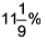QUESTION: 6

DIRECTIONS for questions: Select the correct alternative from the given choices.

The sides of a rightangled triangle are in geometric progression. Find the common ratio, if it is greater than 1.

Solution:

Let the sides of the triangle be a, ar and ar2.

The largest side of a right angled triangle is the hypotenuse.

Hence it must be ar2

By hypotenuse theorem,

a2 + (ar)2 = (ar2)2

a2 + a2r2 = a2r4.

=> a2(1 + r2) = a2r4.

=> r4- r2- 1 =0.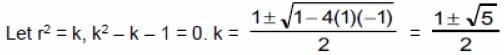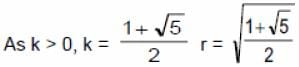QUESTION: 7

DIRECTIONS for questions: Select the correct alternative from the given choices.

A party was attended by twenty five persons. There were a lot of handshakes. Which of the following statements can never be true?

Solution: We consider the choices one by one

(a) A person, say A may have shook hands with 1,3,5 etc people. This is possible.

(b)A person may have shook hands with all the others. This is possible.

(c)If each person has shaken hands with a different number of people, the number of handshakes for the 25 people has to be 0, 1, 2. ....24. But if one person has 0 handshakes, no person can have made 24 handshakes. At the most a person can have made 23 handshakes. This statement can never be true.

QUESTION: 8

DIRECTIONS for questions: Select the correct alternative from the given choices.

A square sheet of paper has a side of 30 cm. Four squares, each of side x‘ ’ cm, are cut away from each of its corners and the remaining sheet is folded into an open cuboid (i.e., without the top face). What is the maximum possible volume (in cc.) of the cuboid formed?

Solution: If the side of each square cut out from the four comers of the square sheet is x cm then the open cuboid formed by folding the rest of the square sheet will be of the dimensions.

Height = x cm and length = breadth = 30 - 2x cm

Now to maximise this volume x(30 - 7x) (30 - 2x) must be the maximum.

If x(30 - 2x)2 is maximum, then 2x(30 - 2x)2 must also be maximum.

Now for any expression of the form x(K - x)n to be the maximum, x and (K - x) must be in the ratio 1 : n.

Hence for 2x (30 - 2x)2 to be maximum 30 – 2x / 2x = 2 / 1

=> x = 5 => 5(30 - 2 X 5)2 = 2000.

QUESTION: 9

DIRECTIONS for questions: Select the correct alternative from the given choices.

Fourteen children are playing a game on a beach. Initially they begin the game by making one huge pile of sand and then take turns to divide the pile into smaller piles. If each child, in his or her turn, can divide an existing pile into only m or n smaller piles, for what value of (m, n) is it not possible to get 14 piles of sand at any stage in the game?

Solution: We can proceed from the options

(1) (m, n) = (2, 5)

As one pile can be divided into 2, the number of piles can increase by steps of 1. It’ll be possible to get 1, 2, 3,4, 5,….piles.

(2)(m, n) = (3, 6)

In any turn, the number of piles can increase by 2 or 5.

∴It can increase by four 2’s and one 5, i.e., by 13. Hence, from 1 to 14.

Hence it is possible in this case also.

(3)(m, n) = (3, 9)

In any turn, the number of piles can increase only by 2 or 8. Hence, starting from 1 pile, we can only get an odd number (any odd number) of piles, but not 14 piles at any stage. Hence, it is not possible in this case.

(4)(m, n) = (5, 6)

In any turn, the number of piles can increase by 4 or 5.

∴ It can increase by two 4's and one 5, i.e. by 13 (say from 1 to 14)

Hence, it is possible in this case.

QUESTION: 10

DIRECTIONS for questions: Select the correct alternative from the given choices.

If log10 a2b = 5 and log10(a / b2) = 8, then find the value of a.

Solution:

Given log10a2b= 5 => 2log10 a + log10 b = 5

Also, logio a/b2 = 8 => log10 a - 2log10 b = 8

Solving for log a, we get log10 a = 18/5

=> a = (10)18 / 5

QUESTION: 11

DIRECTIONS for questions: Select the correct alternative from the given choices.

Two vessels, A and B, contain milk and water respectively. The volume of milk in A and the volume of water in B are in the ratio 4 : 5. Now, x litres of milk from A is poured into B and then y litres of the solution from B is poured into A. If as a result, the concentration of milk in A and that of water in B, both dropped from 100% to 66 x 2/3%, find x : y.

Solution: Let the initial volume of milk in A be 4a.

=> Volume of water in B is 5a.

After the transfer of contents from A to B, the concentration of water in B becomes 66 x 2/3%: i.e., 2/3. Hence, the total volume, must have become 3/2 times the initial (i.e., 5a). Hence, the volume of milk transferred to B = 3 / 2 (5a)- 5a = 5 / 2 a = 2.5a .

∴Milk remaining in A = 4a - 2.5a = 1.5a

Now, the concentration of milk in A and B = 100% and 33 x 1/3%.

If after transferring some quantity of the contents of B to A, the concentration becomes 66 x 2/3, (i.e., mid-way between 100 and 33 x 1/3%, that means that the volume of contents from B and the volume of milk in A were mixed in equal quantities, i.e., 1.5a of milk and water solution was transferred from B to A.

∴ Required ratio = 2.5a / 1.5a = 5 : 3.

QUESTION: 12

DIRECTIONS for questions: Select the correct alternative from the given choices.

The ithterm, ai, of a sequence is given by the product of the firsti prime numbers starting from 7. The sum of the firstj terms of this sequence is denoted by sj. Which of the following is true?

Solution: ai = (7) (11)…..(the product of i primes,) starting from 7.

∴All the a's are odd.

si = ai + a2 +….+ ai

If i is even, si is even and

If i is odd, si is odd

The converses are also true i.e.

If si is even, i is even and if si is odd, i is odd.

∴ (a)is false.

(b)is false. If ai is odd (all as are odd), li could be odd or even.

(c)is true

(d)is false, as if si is odd, i is odd and ai is always odd.

QUESTION: 13

DIRECTIONS for questions: Select the correct alternative from the given choices.

ABCD is a square, of side 7 units, in the co-ordinate plane, such that the origin lies within the square. If AB is parallel to the y-axis and A is at (−4, 2), what are the coordinates of C?

Solution: Since A is in the second quadrant, B must be in the third quadrant (if the origin is to be contained within the square).

Therefore, the coordinates of B must be (-4. -5), since AB is parallel to y-axis and AB= 7.

Also, since AB is parallel to Y axis, BC must be parallel to X axis.

Now, since B is (-4, -6) and BC=7, the coordinates of C must be (3, -5).

QUESTION: 14

DIRECTIONS for questions: Select the correct alternative from the given choices. There are two groups of workers, G1 and G2. There are 30 workers in G1 and 60 in G2. Each worker in G1 works three times as fast as any worker in G2. If all the workers in G1 together ploughed a certain field in half a day, then find the time taken by all the workers in G1 and G2 together to plough a field whose area is 50% more than that of the first field.

Solution: Let the rate at which each worker in G2 works be x units/day

Rate at which each worker in G1 works = 3x units/day

Let the area of the first field be w w = 30(3x)(0.5) = 45x

Area of second field = 1,5w = 67.5x

Let the required time be t days

∴ t[30(3x)+60(x)] = 67.5x

⇒ t = 67.5 / 150 = 9 / 20

QUESTION: 15

DIRECTIONS for questions: Select the correct alternative from the given choices.

Consider two cubes of equal volume. In one of the cubes, the biggest possible sphere is inscribed. The other cube is cut into exactly 64 identical small cubes. In each of them, the biggest possible sphere is placed. The volume of the sphere in the first cube is denoted by V1. The total volume of the spheres in the small cubes is denoted by V2. Find V1 : V2.

Solution: For any cube, the ratio of the volume of the largest possible sphere that can be placed in it to its volume is constant.

As the sum of the volumes of all the small cubes is equal to the volume of the first cube, the sum of the volumes of the spheres in these cubes is equal to the volume of the sphere is the first cube.

∴The required ratio is 1 :1.

QUESTION: 16

DIRECTIONS for questions: Select the correct alternative from the given choices.

Mrs. Ahluwalia spent less than Rs.10,000 to buy some cotton sarees and some silk sarees. If Mrs. Ahluwalia had spent the amount of money she actually spent on cotton sarees to buy silk sarees and vice versa, she could have purchased two more sarees. Find the maximum number of sarees that Mrs. Ahluwalia could have originally purchased, if each cotton saree and silk saree costs Rs.300 and Rs.400 respectively.

Solution: Let the number of cotton sarees and silk sarees originally bought be x and y respectively.

∴300x + 400y< />

Now, if 300x rupees are spent to buy silk sarees, the number of silk sarees that could be purchased is 300x / 400 or 3 / 4x. Similarly if 400y rupees are spent to buy cotton sarees, the number of cotton sarees that could be purchased is 400y / 300 or 4 / 3 y

Given : x + y + 2 = 4 / 3y + 3 / 4x

=>4y-3x = 24. Tabulating the possible values of x and y, we have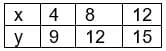The higher values of x and y are ignored as 300x + 400y < 10000.="" hence="" mrs.="" ahluwalia="" could="" have="" bought="" a="" maximum="" of="" 27="" />

QUESTION: 17

DIRECTIONS for questions: Select the correct alternative from the given choices.

How many distinct rectangles of area 900 sq.cm are possible with both their length and breadth being integers, when expressed in cm?

Solution: Area = 900 sq.cm = 22 x 32 x 52. Now the required number of rectangles is nothing but the number of distinct ways in which 900 be written as a product of two numbers i.e., 1 / 2 [(2 + 1) (2 + 1) (2 + 1) + 1 ] = 14.

QUESTION: 18

DIRECTIONS for questions: Select the correct alternative from the given choices.

If S1 is a set comprising ten integers and S2 is a subset of S1 comprising nine elements, which of the following statements are true?

I.The range of S2 can be more than that of S1

II.The median of S2 can be less than that of S1

III.The mean of S2 can be more than that of S1

Solution:

Statement I

S2 is a subset of Si and it has one element less than S1.

If this element was the greatest or the least element of S1, range of S2< range of S1

(Range = Max element -Min element)

If this element was neither the greatest element nor the least element of S1, range of S2 = range of S1.

In any case, range of S2 cannot be > range of S1

Statement I is false.

Statement II

Let S1 = {1,2, 3, 4, 5, 6, 7, 8, 9, 10} and

S2 = {1,2, 3,4, 5,6, 7, 8,9}

Median of S1 = 5th element + 6th element / 2 = 5 + 6 / 2 = 5.5

Median of S2 = 5th element = 5

Statement II is true.

Statement III

Let S1 = {1,2, 3, 4, 5, 6, 7, 8, 9, 10} and

S2 = {2, 3, 4, 5, 6,7, 8, 9, 10}

MeanofS1= 55 / 10 = 5.5 and mean of S2 = 54 / 9 = 6

Statement III is true.

Only statements II and III are true.

QUESTION: 19

DIRECTIONS for questions: Select the correct alternative from the given choices.

Sam and Ram together started a business with initial investments in the ratio of 3 : 4 but Ram left the business aftexr months. If at the end of the year, Sam and Ram shared the profit in the ratio 3 : 2, what is the value of x?

Solution: Since Ram left the business after x months, the profits will be split in the ratio, 3x12 / 3 = 3 / 2 => x = 6.

QUESTION: 20

DIRECTIONS for question: Select the correct alternative from the given choices.

If a Ψb = ab − (a + b) + 1

a π b = LCM (a, b) / a

a Λ b = ab / a + b

what is the value of (6 Ψ 13)π (12 Λ 3)?

Solution:

(6 Ψ 13) = 78 – 19 + 1 = 60

(12 Λ 3) = 36 / 15 = 12 / 5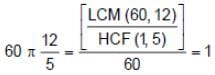QUESTION: 21

Fresh dates contain 80% water by weight while dry dates contain 20% water by weight. What is the weight (in kg) of dry dates available from 300 kg of fresh dates?

Solution:

300 kg of fresh dates contain 240 kg water

=> 60 kg is non-water.

=> 60 kg is 80% of dried grape.

Weight of dried grapes

= 60 / 80% = 60 x 5 / 4 = 75 Kg

QUESTION: 22

In an obtuse triangle, the difference between the obtuse angle and one of the acute angles of a triangle is 20°, while the difference between the two acute angles is 56°. Find the measure (in degrees) of the smallest angle of the triangle.

Solution: Let the obtuse angle be a, and the acute angles be b and c.

Now, given that

a - b = 20 => a = 20 + h and

b - c = 56 => c = b - 56

Also, a + b + c = 180 => (20 + b) + b + (b - 56) = 180

=> 3b = 216 or b = 72 => a = 20 + 72 = 92

and c = 72 - 56 = 16

QUESTION: 23

If x is an integer and x3 − 2(x + 2)2 = 993, find the value of x.

Solution: x3 - 2(x + 2)2 = 993

=> x3 + 23 - 2(x + 2)2 = 1001

=> (x + 2) [x2 - 2x + 4 - 2x - 4] = 1001

=> (x + 2) (x) (x - 4) = 13 x 11 x 7 = 1001

∴ x = 11

QUESTION: 24

In a school, there are three houses, A, B and C. If 170 students leave house B and join house C, the ratio of the number of students in house A and house C would be reversed. Instead, if 40 students leave house A and join house C, then the number of students in house A and house C will be equal. Find the number of students in house A and house C together.

Solution:

Let the number of students in house A be x.

=> the initial number of students in house C be x - 80.

=> x / x – 80 = (x – 80) + 170 / x

=> x2 = (x – 80)2 + 170(x - 80)

=> x2 = x2 - 160x + 6400 + 170x - 13600

=> 10x = 7200

∴ x = 720

∴ The number of students in house A and house C is

x + x - 80 = 2(720) - 80 = 1360.

QUESTION: 25

The product of three numbers is 1620. If the HCF of any two out of the three numbers is 3, find the LCM of the three numbers.

Solution: Let the three numbers be 3a, 3b and 3c. As HCF (3a, 3b)

= HCF (3b, 3c) = HCF (3a, 3c) = 3

=> The LCM of the three numbers = 3abc

The product of the numbers = 27abc = 9(3abc) = 1620

∴ 3abc= 1620 / 9 =180

∴ The LCM of the three numbers is 180.0Use Code STAYHOME200 and get INR 200 additional OFF Use Coupon Code